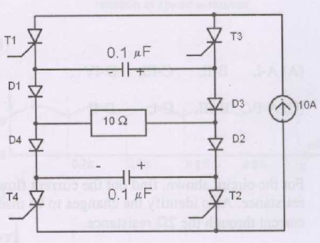## Power Electronics Solved Objective Questions asked in Competitive Exams(GATE, IES)

 An SCR is considered to be a semi-controlled device because [GATE 2009]
(a) It can be turned OFF but not ON with a gate pulse
(b) It conducts only during one half-cycle of an alternating current wave
(c) It can be turned ON but not OFF with a gate pulse
(d) It can be turned ON only during one half-cycle of an alternating voltage wave

 The current Source Inverter shown in figure is operated by alternatively turning on thyristor pairs(T1,T2) and (T3,T4). If the load is purely resistive, the theoretical maximum output frequency obtainable will be [GATE](a) 125kHz
(b) 250kHz
(c) 500kHz
(d) 50kHz

 In the chopper circuit shown, the main thyristor(TM) is operated at a duty ratio of 0.8 which is much larger the communication interval. If the maximum allowable reapplied dv/dt on TM is 50V/μs,what should be the theoretical minimum value of C1? Assume current ripple through L0 to be negligible. [GATE]
(a) 0.2μF
(b) 0.02μF
(c) 2μF
(d) 20μF

 Match the switch arrangements on the top row to the steady-state V-I characteristics on the lower row.The steady state operating points are shown by large black dots. [GATE](a) A-I, B-II, C-III, D-IV
(b) A-II, B-IV, C-I, D-III
(c) A-I, B-II, C-III, D-II
(d) A-IV, B-III, C-II, D-I

 " Six MOSFETs connected in a bridge configuration (having no other power device) MUST be operated as a Voltage Source Inverter (VSI) ". This statement is [GATE 2007]
(a) True, because being majority carrier devices, MOSFETs are voltage driven
(b) True, because MOSFETs have inherently anti parallel diodes
(c) False, because it can be operated both as current source Inverter (CSI) or a VSI
(d) False, because MOSFETs can be operated as excellent constant current sources in the saturation region

 A single phase full - wave half controlled bridge converter feeds an inductive load. The two SCRs in the converter are connected to a common DC bus. The converter has to have a free wheeling diode [GATE 2007]
(b) Because the converter does not provide for free wheeling for high values of triggering angles
(c) Or else the free wheeling action of the converter will cause shorting of the AC supply
(d) Or else if a gate pulse to one of the SCRs is missed, it will subsequently cause a high load current in the other SCR

 A single phase fully controlled thyristor bridge ac-dc converter is operating at a firing angle of 25 degree, and an overlap angle 10 degree with constant dc output current of 20A. The fundamental power factor ( displacement factor) at input ac mains is [GATE 2007]
(a)  0.78
(b)  0.827
(c)  0.866
(d)  0.9

 A three phase fully controlled thyristor bridge converter is used as line commutated inverter to feed 50KW power at 420V DC to a three phase 415V(line), 50Hz as mains. Consider DC link current to be constant. The RMS current of the thyristor is [GATE 2007]
(a) 119.05A
(b) 79.37A
(c) 68.73A
(d) 39.68A

The transfer function of a compensator is given as GC(s)=[(s+a)/(s+b)]
  GC(s) is a lead compensator if
(a) a=1, b=2
(b) a=3, b=2
(c) a=-3, b=-1
(d) a=3, b=1

 The phase of the above lead compensator is maximum at

 A three phase to single phase conversion device employs a 6 pulse bridge cycloconverter. For an input voltage of 200V/phase, the fundamental rms value of output voltage is
(a) 600/π V
(b) 300√3/π V
(c) 300/π V
(d) 600√3 /π V

 A three phase to single phase cycloconverter consists of positive and negative group of converters. In this device one of the two component converters would operate as a

(a) Rectifier if the output voltage VO and output current IO have the same polarity
(b) Inverter if VO and IO have the same polarity
(c) Rectifier if VO and IO are of opposite polarity
(d) Inverter if VO and IO are of opposite polarity
Options:
1) A, D
2) A
3) D
4) C, D

 A 3 phase to 3 phase cycloconverter requires

(a) 18 SCRs for 3-pulse device
(b) 18 SCRs for 6-pulse device
(c) 36 SCRs for 3-pulse device
(d) 36 SCRs for 6-pulse device
Options:
1) A
2) A, D
3) B, D
4) D

 Which of the following statements are correct for cycloconverter?

(a) Step down cycloconverter works on natural commutation
(b) Step up cycloconverter requires forced commutation
(c) Load commutated cycloconverter works on line commutation
(d) Load commutated cycloconverter requires a generated emf in the load circuit.
Options:
1) B, D
2) A, B, C
3) B, C
4)A, B, D

 The functions of connecting a resistor in series with gate-cathode circuit and a zener diode across gate-cathode circuit are, respectively to protect the gate circuit from

(a) Over voltages, over currents
(b) Over currents, over voltages
(c) Over currents, noise signals
(d) Noise signals, over voltages

5:05 PM